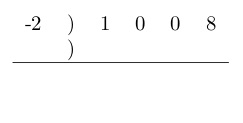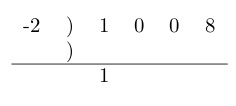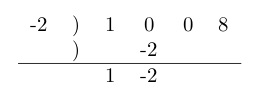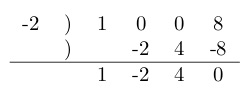# Synthetic Division

You’re not likely to get a CLEP question that requires you to do synthetic division, but you quite likely could get a question for which you need to divide polynomials. Synthetic division is an algorithm that allows you to divide polynomials faster than you can with regular old polynomial long division (pretty much because you don’t have to keep writing x, x, x . . . .). You can do it even if you never mastered polynomial long division.

Synthetic division works when you divide by a binomial: , where  is some real number.

Here’s how synthetic division works:

Say your problem is . Then  and

• Set up something that looks like this:• Write in  where  is shown below. To the right of that write in the coefficients of the  terms. The coefficient of  is 1. There is no  or  term, but treat those as if they exist, with coefficients of 0. And the constant term is 8. The setup should look like this:• From the list of coefficients, copy the one farthest to the left – that’s 1 – to directly below itself just below the horizontal line.• Multiply that 1 by the -2 all the way over on the left. Write the result in just above the horizontal line, directly below the next term in the coefficient list (the first 0 in this case).• Add that 0 to the number you just wrote under it, and write the sum below the horizontal line.• Multiply the -2 all the way on the left by the -2 you just wrote under the horizontal line. Write the product under the next entry on the coefficient list, in this case the next 0. Add the number you just wrote to the number above it, and write the sum under the horizontal line. Keep going like that until you are out of numbers in the coefficient line.The numbers below the line represent the answer. The expression is one degree lower than the one you divided. Since in this case the expression you divided is third degree, the answer is second degree. The first term is the coefficient of , the second term is the coefficient of , the third term is a constant term, and the fourth is the remainder.